## Thursday, 7 August 2014

### chapter- 19 Worked Out Examples

 Example: 17
 Find the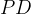$PD$ of the number of doublets in three throws of a pair of dice.
 Solution: 17
In three throws, we can obtain either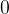$0$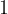$1$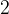$2$, or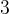$3$ doublets. Denote this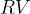$RV$by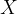$X$.
Now, doublets can be obtained in$6$ ways, namely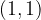$(1, 1)$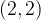$(2, 2)$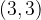$(3, 3)$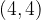$(4, 4)$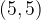$(5, 5)$ and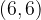$(6, 6)$, out of a total possible ways of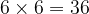$6 \times 6 = 36$. Thus, the probability of obtaining a doublet is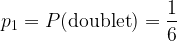${p_1} = P({\rm{doublet}}) = \dfrac{1}{6}$ and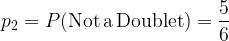${p_2} = P({\rm{Not\, a \,Doublet}}) = \dfrac{5}{6}$
Now, let us obtain the probabilities for the different values of$X$.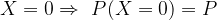$X = 0 \Rightarrow\,\, P(X = 0) = P$ {No doublets in all three throws}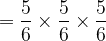$= \dfrac{5}{6} \times \dfrac{5}{6} \times \dfrac{5}{6}$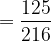$= \dfrac{{125}}{{216}}$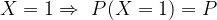$X = 1 \Rightarrow \,\,P(X = 1) = P$ {A doublet exactly once}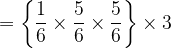$= \left\{ {\dfrac{1}{6} \times \dfrac{5}{6} \times \dfrac{5}{6}} \right\} \times 3$ {Why is there the factor of$3$?}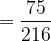$= \dfrac{{75}}{{216}}$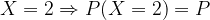$X = 2 \Rightarrow P(X = 2) = P$ {Exactly two doublets}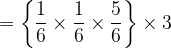$= \left\{ {\dfrac{1}{6} \times \dfrac{1}{6} \times \dfrac{5}{6}} \right\} \times 3$ (why a factor of$3$ again?)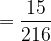$= \dfrac{{15}}{{216}}$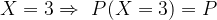$X = 3 \Rightarrow\,\, P(X = 3) = P$ {Doublets in all three throws}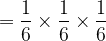$= \dfrac{1}{6} \times \dfrac{1}{6} \times \dfrac{1}{6}$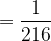$= \dfrac{1}{{216}}$
Thus, the$PD$ isNote the (obvious) fact that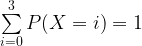$\sum\limits_{i = 0}^3 {P(X = i) = 1}$
 Example: 18
 Find the$PD$ of the number of Heads in four tosses of a coin.
 Solution: 18
Let$X$ denote the number of Heads in the four tosses
Verify the following probabilities: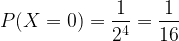$P(X = 0) = \dfrac{1}{{{2^4}}} = \dfrac{1}{{16}}$$P(X = 1) = {\,^4}{C_1} \times \dfrac{1}{{{2^4}}} = \dfrac{1}{4}$$P(X = 2) = {\,^4}{C_2} \times \dfrac{1}{{{2^4}}} = \dfrac{3}{8}$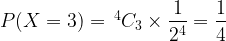$P(X = 3) = {\,^4}{C_3} \times \dfrac{1}{{{2^4}}} = \dfrac{1}{4}$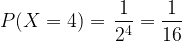$P(X = 4) = \,\dfrac{1}{{{2^4}}} = \dfrac{1}{{16}}$
Thus, the$PD$ is